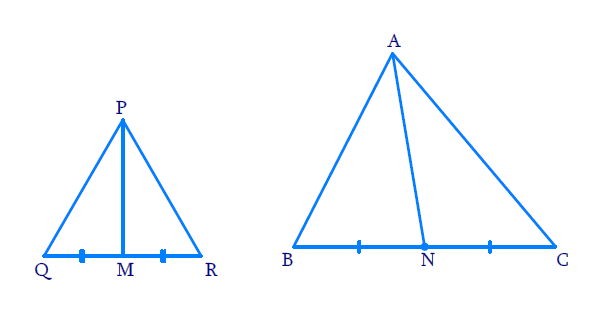# Ex.6.4 Q6 Triangles Solution - NCERT Maths Class 10

Go back to  'Ex.6.4'

## Question

Prove that the ratio of the areas of two similar triangles isequal to the square of the ratio of their corresponding medians.

Diagram## Text Solution

Reasoning:

As we know,if one angle of a triangle is equal to one angle of the other triangle and the sides including these angles are proportional, then the two triangles are similar.And we know that the ratio of the areas of two similar triangles is equal to the square of the ratio of their corresponding sides.

Steps :

In $$\Delta PQR$$, $$PM$$ is the median and, in $$\Delta ABC$$ $$AN$$ is the median

$$\Delta P Q R \sim \Delta A B C(\text { given })$$

\begin{align} \angle PQR&=\angle ABC \qquad\qquad\dots\dots\dots\left( 1 \right) \\\angle QPR\text{ }&=\angle BAC\text{ }\qquad\qquad\dots\dots\dots\left( 2 \right) \\ \angle QRP\text{ }&=\angle BCA\text{ }\qquad\qquad\dots\dots\dots\left( 3 \right)\\\text{and}\; \frac{PQ}{AB}&=\frac{QR}{BC}=\frac{RP}{CA}\qquad\dots\dots\dots(4)\end{align}

($$\because$$ If two triangles are similar, then their corresponding angles are equal andcorresponding sides are in the same ratio)

\begin{align}\frac{{Area\,of\,\Delta PQR}}{{Area\,of\,\Delta ABC}} = \frac{{{{(PQ)}^2}}}{{{{(AB)}^2}}} = \frac{{{{(QR)}^2}}}{{{{(BC)}^2}}} = \frac{{{{(RP)}^2}}}{{{{(CA)}^2}}} \end{align} [from Theorem 6.6] .....(5)

Now In $$\Delta PQM$$ and $$\Delta ABN$$

$$\angle PQM = \angle ABN$$ (from 1)

And \begin{align}\frac{{PQ}}{{AB}} = \frac{{QM}}{{BN}}\end{align}

$\left[ {\,\,\,\frac{{PQ}}{{AB}} = \frac{{QR}}{{BC}} = \frac{{2QM}}{{2BN}};M,{\rm{ }}N{\text{ }}mid{\text{ }}points{\text{ }}of{\rm{ }}QR{\text{ }}and{\text{ }}BC} \right]$

$$\Rightarrow \Delta PQM \sim \Delta ABN$$ [$$SAS$$similarity]

$\Rightarrow \,\,\frac{{Area\,of\,\Delta PQM}}{{Area\,of\,\Delta ABN}} = \frac{{{{(PQ)}^2}}}{{{{(AB)}^2}}} = \frac{{{{(QM)}^2}}}{{{{(BN)}^2}}} =\frac{{{{(PM)}^2}}}{{{{(AN)}^2}}}\left[ {\,\,theorem\,\,6.6} \right]$

from(5) and (6)

\begin{align}\frac{Area\,of\,\Delta PQR}{Area\,of\,\Delta ABC}=\frac{{{(PM)}^{2}}}{{{(AN)}^{2}}}\end{align}

Learn from the best math teachers and top your exams

• Live one on one classroom and doubt clearing
• Practice worksheets in and after class for conceptual clarity
• Personalized curriculum to keep up with school